[BACK]Computer Modeling in Engineering & SciencesDOI: 10.32604/cmes.2021.015673

ARTICLE

Code Transform Model Producing High-Performance Program

1Department of Computer Science and Information Engineering, National University of Kaohsiung, Kaohsiung, Taiwan
2Department of Fragrance and Cosmetic Science, Kaohsiung Medical University, Kaohsiung, Taiwan
*Corresponding Author: Bao Rong Chang. Email: brchang@nuk.edu.tw
Received: 04 January 2021; Accepted: 21 May 2021

Abstract: This paper introduces a novel transform method to produce the newly generated programs through code transform model called the second generation of Generative Pre-trained Transformer (GPT-2) reasonably, improving the program execution performance significantly. Besides, a theoretical estimation in statistics has given the minimum number of generated programs as required, which guarantees to find the best one within them. The proposed approach can help the voice assistant machine resolve the problem of inefficient execution of application code. In addition to GPT-2, this study develops the variational Simhash algorithm to check the code similarity between sample program and newly generated program, and conceives the piecewise longest common subsequence algorithm to examine the execution's conformity from the two programs mentioned above. The code similarity check deducts the redundant generated programs, and the output conformity check finds the best-performing generative program. In addition to texts, the proposed approach can also prove the other media, including images, sounds, and movies. As a result, the newly generated program outperforms the sample program significantly because the number of code lines reduces 27.21%, and the program execution time shortens 24.62%.

Keywords: Newly generated programs; GPT-2; predetermined generative programs; variational Simhash algorithm; piecewise longest common subsequence

1  Introduction

As Google DeepMind developed alpha Go in London in 2014, it defeated all other Go masters. Since then, the research of artificial intelligence  has been increasing again. The performance of deep learning neural networks can surpass that of traditional neural networks. Not only can it automatically intercept features and reduce target errors, the most widely studied models with generation topics as the research topic are Long Short-Term Memory (LSTM)  and Generative Adversarial Network (GAN) . LSTM can roughly generate texts. However, LSTM only refers to preceding words that appear and predicts what word appears next. LSTM cannot use the input data to regenerate articles with similar topics. GAN performs better-imitating patterns than imitating text. Even though deep learning is relatively mature, human beings are often unsatisfied. As a result, the most research direction for machines to imitate human beings is to develop language that imitates humans.

An emerging issue concerning artificial intelligence has inspired machine learning or deep learning in recent years. With hard efforts and development, the current technology can make the machine understand different tasks, such as Tesla's NoA, Apple's Siri, Amazon Echo & Alexa, and Google Home. At present, there are quite a lot of brands and types of voice assistant machines in the world, and they use their existing programs for human-computer interaction in response to user requests. However, some voice assistant machines have encountered the problems, that is, the engine may not be able to answer the questions correctly , or the existing programs have low execution efficiency . Nevertheless, we try to resolve the problem of inefficient execution and think about the machine transforming a current program into a newly generated program that can run a higher efficiency program and produce the correct execution result?

The natural language processing mechanism involves understanding and generating. The Natural Language Toolkit (NLTK)  has been developed for the English Natural Language Segmentation Model in English's natural language development. Through training and using this model, it can segment natural language sentences into words, and actual words can realize the sentences. The most famous English model is GPT-2 , the second generation of Generative Pre-trained Transformer. GPT-2 is imitation research in the field of artificial intelligence developed by OpenAI LP . Transformer model  acts like human beings, and thus it can generate fake news. These tools function as the basis of human language imitation and play a key role in applications. The most common computer programming that runs with the tools mentioned above is Python . With GPT-2, based on predetermined generative programs in statistics , the proposed procedure can produce many generated programs appropriately to respond to the inquiry.

The motivation of this study is to use machines to generate usable programs through GPT-2. The purpose is to solve the problem of low execution efficiency in some voice assistant machines. The goal of this paper is thus to speed up the execution performance in applications. The following paragraphs of this paper are arranged as follows. In Session 2, This section will introduce the related work of word segmentation processing and language generation models. The way to system implementation is given in Session 3. The experimental results and discussion will be obtained in Session 4. Finally, we drew a brief conclusion in Session 5.

2  Related Work

This study has developed efficient code to improve the program execution performance in applications and used the following key technologies: Anaconda (Data Science Platform with Virtual Environment Conda), Tensorflow (Dataflow and Differentiable Programming), NLTK (English Text Segmentation), GPT-2 (Text Generating Model), Variational Simhash (Cosine Text Similarity Algorithm), and Piecewise Longest Common Subsequence to achieve the goal of this paper.

2.1 Language Generation Model--Generative Pre-Training 2

The second generation of Generative Pre-Training Transformer (GPT-2) is an unsupervised  language model , released by OpenAI in 2019. Researchers believe that the language model of unsupervised learning is a general language one. Furthermore, GPT-2 proves that the model is not trained for any specific task to predict the next word as the training target. Use sentence database WebTex  for data training, which contains 8 million web pages as the training data. These web pages are part of Reddit  and are more than 40 GB. Compared with other deep learning algorithms for generating texts, Long Short-Term Memory (LSTM) or Generative Adversarial Network (GAN) is smoother. Its main advantage is that the code is English the training model is easier to understand. The traditional Transformer model is composed of Encoder and Decoder, called the Transformer architecture stack, as shown in Fig. 1. This model solves the problem of machine translation.Figure 1: Transformer architecture stack

In many subsequent studies, the Transformer architecture removes either Encoder or Decoder, uses only one Transformer stack, and provides a large amount of training text and machine equipment. GPT-2 is composed of the Decoder architecture according to the Transformer model. As shown in Fig. 2, the stacking height is the size difference of various GPT-2 models. Currently, there are four sizes of models: GPT-2 Small, GPT-2 Medium, GPT-2 Large, and GPT-2 Extra Large .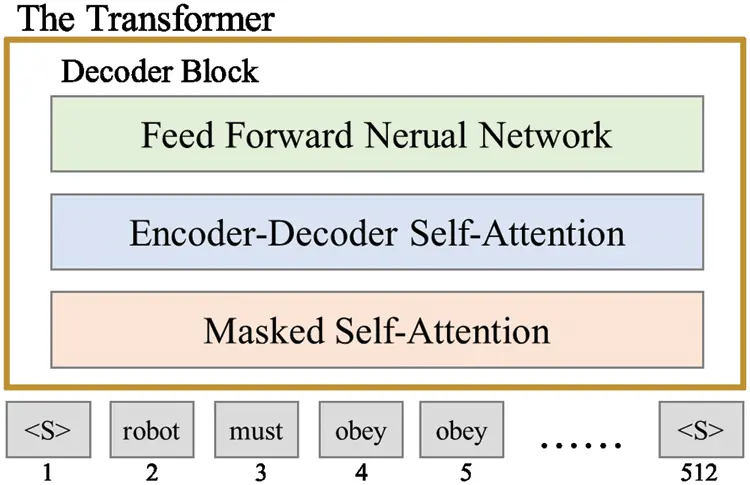Figure 2: GPT-2 decoder architecture

2.2 Predetermined Generative Programs

This part is to determine how many generated programs at least produced by GPT-2, having the pass ratio of over 90% of code similarity . Technically speaking, corresponding to a single sample program, we first have to count the number of generated programs whose pass ratios are over 90% of code similarity, and then we can further calculate the percentage ρi as shown in Eq. (1). Among them, Nsi is the total number of generated programs produced by GPT-2, corresponding to a single sample program, in which xsi is the number of generated programs whose pass ratio of code similarity is more than 90%. After all of the percentages mentioned above are obtained, Eq. (2) can give an average percentage ρ of the generated programs having the pass ratio of more than 90% where t represents the total number of sample programs. After that, we have to determine how many misjudgments are there in xsi, and then calculate the percentage of misjudgments ρmi, as shown in Eq. (3), where xmsi stands for the number of misjudgments within the generated programs having the pass ratio of over 90%. Here, the average percentage of misjudgments ρm can be obtained, as shown in Eq. (4).

ρi=xsiNsi,i=1,2,,t(1)

ρ=i=1tρit(2)

ρmi=xmsixsi,i=1,2,,t(3)

ρm=i=1tρmit(4)

Next, we will count the number of the generated programs ysi that are generated by GPT-2, corresponding to a single sample program, having the pass ratios of below 90%, and then we can further calculate the percentage μi as shown in Eq. (5). After all of the percentages mentioned above are obtained, Eq. (6) can give an average percentage μ of the generated programs having the pass ratio less than 90%. After that, we can determine how many misjudgments are there in ysi, and then calculate the percentage of misjudgments μmi, as shown in Eq. (7), where ymsi represents the number of misjudgments within the generated programs having a pass ratio of below 90%. Here, the average percentage of misjudgments μm can be obtained, as shown in Eq. (8).

μi=ysiNsi,i=1,2,,t(5)

μ=i=1tμit(6)

μmi=ymsiysi,i=1,2,,t(7)

μm=i=1tμmit(8)

Finally, we add up all of the generated programs produced by GPT-2, corresponding to all of the sample programs, to be represented by Ng as shown in Eq. (9). After obtaining Ng,q,qm,u,andum as mentioned above, Eq. (10) can calculate an average probability Pgq  of the generated programs having a pass ratio of over 90%. We assume that there are j programs with the pass ratio of code similarity of more than 90%. So P(KjPgt) standing for the probability of the pass ratio of code similarity more than 90% for these j programs is real, as shown in Eq. (11) , where P(KjPgt) means the probability of at most j programs having the pass ratio of code similarity over 90% in the generated programs, and Kj represents j programs having the pass ratio of code similarity over 90% in the generated programs. According to statistics, we know that the probability of j programs having the pass ratio of code similarity over 90% is P(KjPgt). We can deduce the least programs must be generated to guarantee j programs are having the pass ratio of code similarity of more than 90%, as shown in Eq. (12), where N is the total number of programs to be generated.

Ng=i=1tNsi(9)

Pgq=Ngq(1qm)+NguumNg(10)

P(KjPgt)=P(KjPgt)Pgt(11)

NP(KjPgt)Kj(12)

Let's take 4 sample programs as an example. Corresponding to each sample program, GPT-2 generates 500 programs respectively and then counts how many programs have the pass ratio of code similarity more than 90% among the 500 programs. For all of the generated programs in 4 cases, we further check each generated program whether its pass ratio of code similarity is more than 90%, and finally obtain the average percentage to be 3%. After that, we compile all of the generated programs having the pass ratio of code similarity over 90%, and some of them are a failure. After judging the number of misjudgments, we can calculate the individual percentage and confirm the average percentage of misjudgments is 1%.

On the other hand, we judge how many of these 500 programs, corresponding to a single sample program, have the pass ratio of code similarity less than 90%, and then calculate the individual percentage for every case. After that, for all 4 cases, we can find the percentage is 97% among the generated programs having the ratio of code similarity less than 90%. Furthermore, we check the number of misjudgments among them and achieve the average percentage of misjudgments is 2%.

According to statistics, we can find that the average probability is 4.91% for generated programs having the pass ratio of code similarity over 90%. We want to know how many programs need to be generated to guarantee five programs having the pass ratio of code similarity over 90%. Those mentioned above, the average probability can be substituted into Eq. (12) to obtain the answer. As a result, at least 100 generated programs must be produced to guarantee 5 of them having the pass ratio of code similarity of more than 90%.

2.3 Program Performance Evaluation

One of the topics in this study is to evaluate the performance improvement of the keyword-enabled generating program from the code transform model. In other words, this study evaluates the execution performance of generated program produced from GPT-2 and the sample program quantitatively. The main performance evaluation includes comparing the number of code lines between the generated program and the sample program and the execution speed. It brings two experiments to apprehend how much performance would be improved in program execution. The performance evaluation has set two indicators (a) the reduction of the percentage of code lines and (b) the shortening of the percentage of program execution time. Reducing the percentage of the program lines of δρ is shown in Eq. (13). The ρs and ρg stood for the number of code lines for sample program and generated program on average, respectively. The reduction of program execution time percentage δτ is shown in Eq. (14). The τs and τg represent the execution time for sample program and generated program on average, respectively.

δρ=(1ρgρs)×100%(13)

δτ=(1τgτs)×100%(14)

3  Research Method

The goal of this study is to achieve the automatic generation of usable programs by human-computer interaction. Thus, propose the newly generated program system where the program is not aimlessly generated. Instead, users must enter sentence inputs into the computer. With three steps, Retrieval, Transformation, and Verification, the proposed system can generate the programs the users require, as shown in Fig. 3. The subject of this study will focus on the development of word segmentation and keyword selection from natural language sentences, code transform model, and code similarity checking together with output conformity examining that are working on a set of GPU cluster systematically. The process is divided into model generation stage and model use stage. The former is further divided into the model training phase and model testing phase. It is expected that the system can achieve the goal of automatic generating programs through human-computer interaction.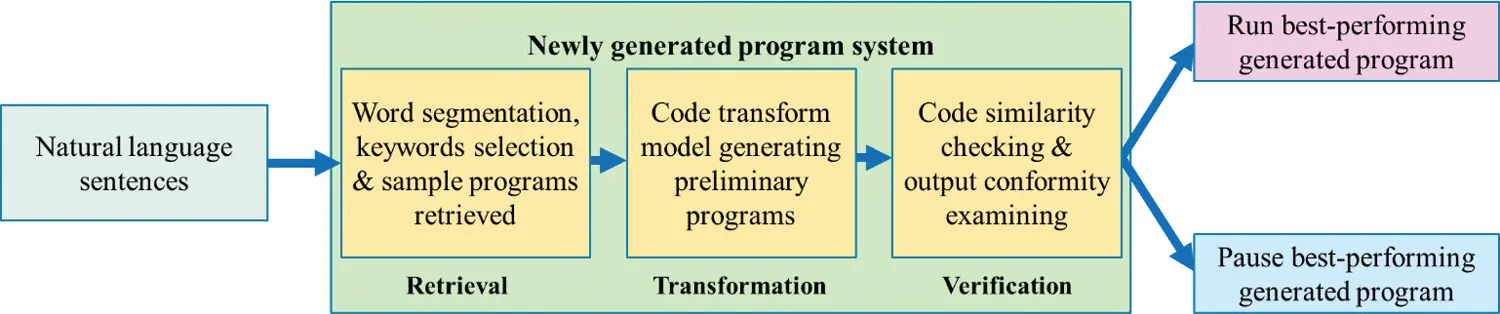Figure 3: Natural language generating program process

3.1 System Architecture of Program Generation

This part introduces the natural language toolkit NLTK and Generative Pre-trained Transformer 2 (GPT-2) to establish a code transform process . This process can produce the newly generated program with high efficient execution. This system uses English natural language sentences as input and is divided into two stages: the model generation stage and model use stage. In the model generating stage, the model will be trained initially after the users enter spoken sentences using text or voice. Then the trained model will be tested to check whether the test pass ratio in generated programs can exceed the predetermined pass ratio. If so, we are looking for the best-performing generated program within the passed-programs and treating it as a pocket program where this useable model's status directly moves to the model use stage. After the users enter a spoken sentence in text or voice in the model use stage, the system will first search for a pocket program generated earlier. If not, the system will get back to the model generating stage and starts its model training process.

The model generation stage includes the training phase and test phase, and their architectures are shown in Figs. 4 and 5. The training phase consisted of four units: word segmentation unit, searching for example programs unit, generating program model unit, and generating program unit. The inputs/outputs during the process are related to natural language sentences, keywords, example programs, program models, preliminary programs, qualified programs, and pocket programs. Users can directly enter Natural language sentences into NLTK. First, the NLTK tool model is used to perform word segmentation and then make keywords selection. Next, we can search for the sample program corresponding to the keywords in the semantic database built in the XAMPP  cloud server, and after that, send the sample program into GPT-2 for the first pass in model training. GPT-2 uses a sample program to train and produces the generative model. After the first pass, the generative model feedback to GPT-2 for the second pass in program generating, where the automatic generation of many preliminary programs. The preliminary programs are obtained, followed by the verification process in three units: test unit, verification unit, and storage unit. The Simhash algorithm checks the code similarity between every preliminary program and the test unit's sample program. The preliminary program with the similarity pass ratio higher than the predetermined one (90%) and successfully compiled with Python is considered a qualified program. When it goes to the verification unit, we proceed LCS conformity comparison between the sample program's execution output and each qualified program, leaving the ones having a pass ratio higher than the predetermined conformity level (95%). Finally, we choose the qualified program having the highest pass ratio as the pocket program, within the ones.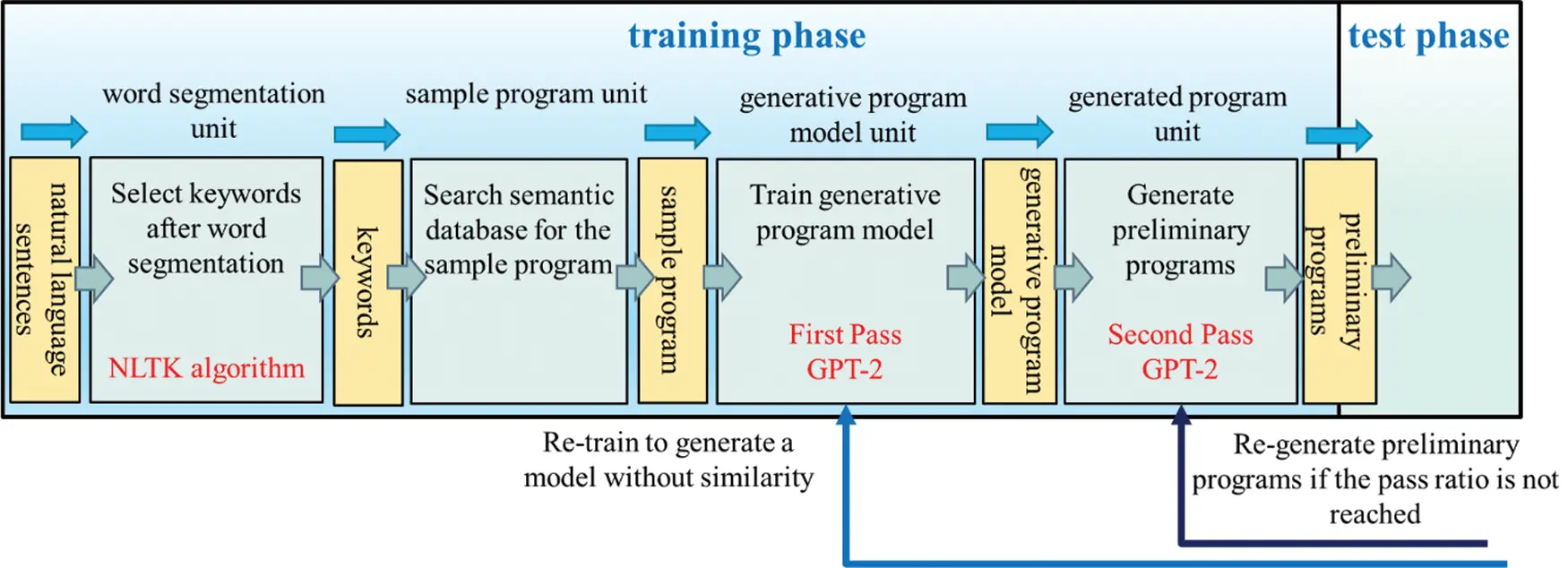Figure 4: Model generation stage—training phase architecture diagramFigure 5: Model generation stage—test phase architecture diagram

After the word segmentation, NLTK can retrieve the selected keywords from the semantic database, and the process jumps directly to the model use stage. Its architecture is shown in Fig. 6. The use stage consisted of five units: word segmentation unit, searching program model unit, generating program unit, evaluation unit, and storage unit. The word segmentation unit allows users to enter natural language sentences into NLTK tool as mentioned above to perform word segmentation and make keyword selection. It will then use the keywords to search the semantic database for a program model or a pocket program that should be chosen and saved in the database earlier. If not, it will retrieve the corresponding generative model, and it then gets to GPT-2 and generates the preliminary programs. Finally, the subsequent verification for the similarity mentioned above and LCS comparison will determine the proper pocket program.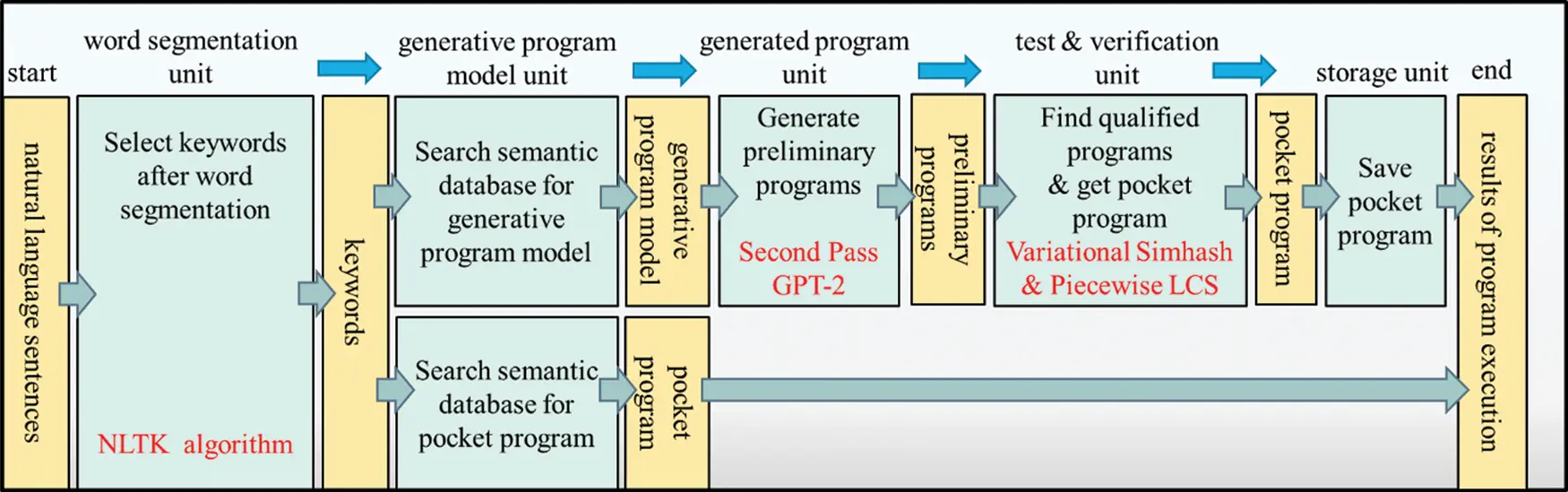Figure 6: Model use stage architecture diagram

3.2 Variational Simhash Algorithm

Since traditional Hash  maps a string into a significant value that cannot be used to measure the similarity of two distinct strings, Simhash  itself belongs to a locally sensitive Hash. Its main idea is to reduce dimensionality, convert a high-dimensional feature vector into an f-bit fingerprint , and determine the similarity of two distinct strings by calculating the Hamming Distance  of two fingerprints. The smaller the Hamming distance, the higher the similarity. Generally speaking, the TF-IDF algorithm is valid for the traditional Simhash algorithm's weighting method to acquire its weighting value. Still, this algorithm is only suitable for general article text comparison, not for a program's code. Therefore, we modified the Simhash algorithm for the code of a program. We first classify the code into reserved and non-reserved words and then find the weight value according to the table look-up or assigning the weight value. For example, the reserved words such as “for”, “while”, and “if” are very similar, and we assign a higher weight value in the look-up table. In contrast, the non-reserved words such as operands and functions give the variable a low weight value.

The overall process is shown in Fig. 7, which includes word segmentation, hash calculation, table look-up weighting, merging, and dimensionality reduction. Word segmentation obtains N-dimensional feature vectors (64-dimensional default) for the text's word segmentation; Hash performs the Hash calculation on all the obtained feature vectors. Table look-up weighting means looking for numerical values to weigh all of the obtained feature vectors through looking up a table as shown in Tab. 1. Merging refers to the accumulation of all the obtained vectors. Dimensional reduction replaces the accumulated result greater than zero to one and less than zero to zero. It obtains a text fingerprint, as shown in Fig. 8, and finally calculates the Hamming distance between two text fingerprints.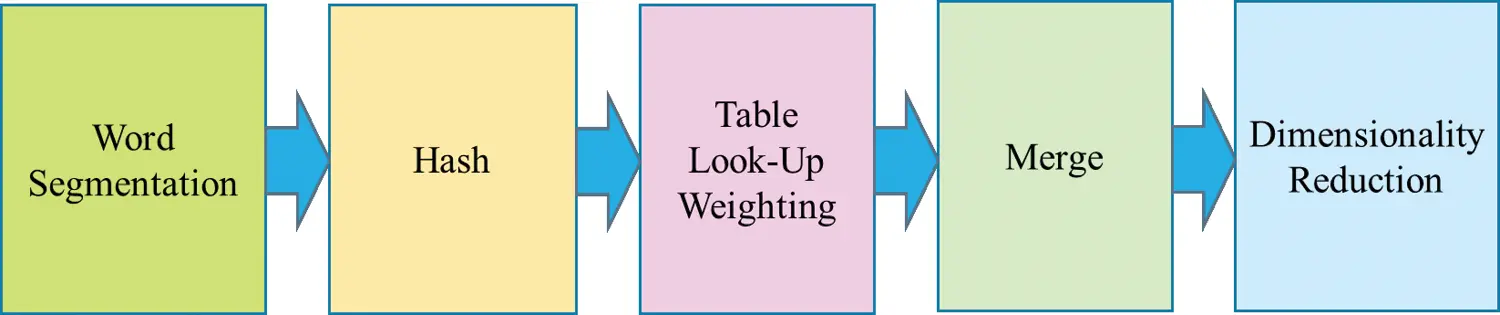Figure 7: Variational Simhash process flow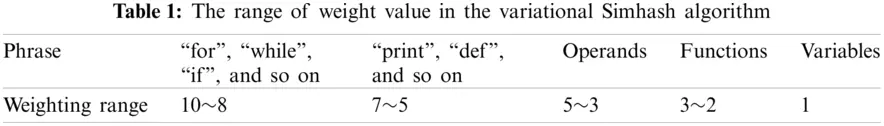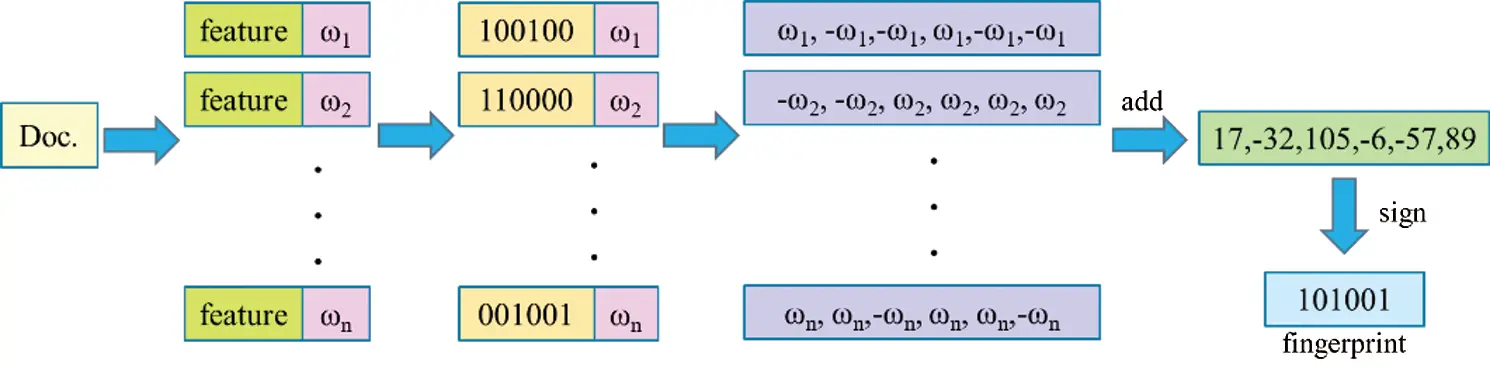Figure 8: Calculate fingerprint

According to the information theory, the Hamming distance between two equal-length character strings is the number of different characters corresponding to the two characters. The Hamming distance is the number of characters that need to replace to convert one string to another character of a fixed length. Moreover, the Hamming distance is a measure of the character vector space that maintains non-negative, unique, and symmetrical. In Hamming distance on Eq. (15) , dHam is the Hamming distance between objects i and j, and k is the index of the corresponding bit reading y in the total number of bits n. In Eqs. (16) and (17), [bi,kbj,k] is the value of 1 or 0 given by the logical value True or False determined according to the internal condition bi,kbj,k. The Hamming distance itself gives the number of mismatched outcomes between bits at the kth position.

dHam=k=0n1[bi,kbj,k](15)

[bi,kbj,k]=1ifbi,kybj,kisTrue(16)

[bi,kbj,k]=0ifbi,kbj,kisFalse(17)

Suppose you measure the similarity of two strings using Hamming distance. In that case, the similarity can be converted into a pass ratio as a metric to describe how much it matches the original object's content. According to the Hamming distance dHam and the total number of bits n, the qualification (pass) ratio γ on Eq. (18) can be obtained.

γ=(1dHamn)×100%(18)

This study used two simple codes of test1  and test2  to test by the method of trial and error and found the appropriate range of weight value as given in Tab. 1. Given appropriate weights based on that, the code similarity between two distinct programs increases to 90% on average compared to the traditional one.

3.3 Piecewise Longest Common Subsequence–PLCS

The Longest Common Subsequence (LCS)  is the problem of finding the longest common subsequence in all sequences in a sequence set (usually two sequences). The Longest Common Subsequence is different from the Longest Common Substring because the subsequence of LCS does not need to occupy consecutive positions in the original sequence. To solve the LCS problem, we cannot use the brute force search method. We need to use dynamic programming to find the LCS and backtracking strategy's length to find the actual sequence of the LCS. However, this study analyzed the traditional LCS computation efficiency and found that when the length of the ASCII or binary code is very long, it will take a long time to complete the conformity comparison. Therefore, we designed the piecewise LCS algorithm called PLCS to improve the execution speed effectively. Once the code of a program is converted into ASCII or binary code, the code will be divided into many of a fixed length of a segment, and then the LCS computation is performed a single segment at a time. After completing each segment of LCS computation, clear the memory, leaving only the result of that segment of LCS. Finally, each segment of LCS adds up to become an overall LCS.

We assume that a piecewise segmented string z = <z1, z2,…, zh> is the PLCS of two piecewise segmented strings xk = <x1, k, x2, k,…, xm, k> and yk = <y1, k, y2, k,…, yn, k>, and we observe that if xm, k = yn, k, then zh, k = xm, k = yn, k, and zh -- 1, k is the LCS of xm -- 1, k and yn -- 1, k; If xm, k ≠ yn, k, then zh is the LCS of xm -- 1, k and yn -- 1, k, or the LCS of xm -- 1, k and yn, k. Therefore, the problem of solving LCS becomes two sub-problems of recursive solution. However, there are many repeated sub-problems in the above-mentioned recursive solution method, and the efficiency is low. The improved method uses space instead of time and uses an array to store intermediate states to facilitate subsequent calculations. Therefore, using the two-dimensional array ck[i,j],k=1,2,,p, where k represents a serial number of the kth segmented string after string segmentation and the number of piecewise segmented strings is p, to record the LCS lengths of two piecewise segmented strings <x1, k, x2, k,…,xi, k> and <y1, k, y2, k,…, yj, k> where the state transition can be obtained on Eq. (19). Afterward, we add up all of the piecewise segmented LCSs to obtain the final LCS on Eq. (20) where imax,k and jmax,k stand for the last index of <x1, k, x2, k,…,xi, k> and <y1, k, y2, k,…, yj, k> segmented strings, respectively, at the kth segmented string.

ck[i,j]={0,i=0,j=0,ork=0ck[i1,j1]+1,i,j,k > 0andxi,k=yj,kmax(ck[i,j1],ck[i1,j]),i,j,k > 0andxi,kyj,k,k=1,2,,p(19)

c=k=1pck[imax,jmax](20)

Use the longest common subsequence to measure the similarity of two programs’ execution results . The similarity is renamed text conformity to illustrate the degree of conformity of the two programs’ respective output results. First, convert the output results of individual programs into ASCII code or binary code, then store them into arrays a and b individually, and then calculate the c array according to the longest common subsequence. Here, the length of the a, b, and c arrays are recorded as |a|, |b| and |c|. The length of the arrays mentioned above is substituted into the formula Eq. (6) to obtain the conformity between texts. Here, the lengths of arrays a, b, and c are denoted as |a|, |b| and |c|, and the length of the above array is substituted into Eq. (21) to obtain LCS conformity denote f.

f=2|c||a|+|b|×100%(21)

This study used four example programs to test by the method of trial and error, namely the article text , the graphic image , the voice signal , and the video signal , where we divided the length of a segment into 10, 100, 1000, 5000, 10,000, and 50,000 ASCII or binary codes, and then performed LCS computation. The time spent on LCS computation is shown in Tab. 2. As a result, we found that in the segment of 10,000 codes, on average, they can complete LCS calculation as fast as possible, and it can increase the execution speed to 51% compared to the case of none of the segments.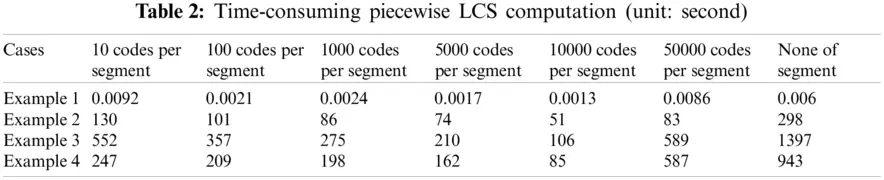3.4 Similarity of Multimedia Signal

Multimedia is the field concerned with the computer-controlled integration of text, graphics, images, sound/audio, animation, video, and other forms. Different types of media have different content and format, corresponding content management and information processing methods are also different, and the storage capacity of information is also very different. In terms of sentence similarity, generally speaking, some distances are used for text comparison, such as Euclidean distance, Manhattan distance, Mahalanobis distance, etc. The smaller the distance, the greater the similarity.

Generally speaking, using the Hash algorithm  to measure the image similarity. By obtaining the hash value of each picture and comparing Hamming distance of the hash value of two images, we can measure whether the two images are similar—the more similar two images, the smaller Hamming distance of the hash value of two images. The comparison of sounds is usually based on some characteristics , such as frequency, tone… etc. After extracting these characteristics, perform the comparison of characteristics to check which features are the differences. As for the comparison of videos, the usual method is to divide the video into frames to make one by one for picture comparison, detect the object in the picture, track the object's position in each image, draw the trajectories, and compare them . Even though there are a few ways to compare different forms of media, this study adopts a single and effective LCS method to compare multimedia information's conformity, which is suitable for various forms of media, including text, pictures, sounds, movies, animations, etc. The following describes the methods of comparing the conformity of various media information one by one.

In terms of text information comparison, first converted the content of the sentences produced by the two programs’ execution into ASCII Code. Then the LCS algorithm is used to compare the ASCII Code of the two sentences. The method for comparing the information of two pictures is to convert the contents of the pictures produced by executing the two programs into binary codes and then using the LCS algorithm to compare the two images’ binary codes. As for the voice information comparison, we first extract some specific characteristic values from two voices produced by the two programs’ execution. Then draw these characteristic values into a picture individually, convert the content of each image into binary codes, and finally compare the binary codes of two images by LCS algorithm. Especially in the calculation of video information comparison, we first use ImageAI's Yolo v3  model to detect the video's object. We then track the object's moving, which is to capture the object's coordinates every second in continuous motion. After that, we draw all the coordinates as a trajectory map. Then, after converting these trajectory maps into binary codes, the LCS algorithm is used to compare the two sets of binary codes.

3.5 Recipe of Hardware and Software

In Fig. 9, a high-level GPU cluster architecture is used for rapid model training to reduce the processing time spent on traditional CPU training models. For the generative program, we choose the code transform model GPT-2. For word segmentation and keyword retrieval, we select NLTK toolkit. The variational Simhash algorithm checks code similarity. The piecewise longest common subssequence algorithm examines the conformity of two different program execution results. All of the tools must build in the cloud environment to execute most applications and generate programs. Therefore, this study uses open-source packages to establish a run-time environment, as listed in Tab. 3.

In GPU cluster architecture, Used two Nvidia brand GPU P100 and two RTX2080Ti. Four GPU cluster workstations are connected through a high-speed local network to accelerate the calculation . The proposed cluster has high availability, reliability, and scalability to form a cloud site or an edge computing. Each workstation server transmits data through a high-speed network QPI, and uses a hardware interface PCIe x16 channel to connect the CPU and GPU. The GPU link uses NVLink  developed by Nvidia to allow four GPUs to share memory using the point-to-point structure and serial transmission. Not only between the CPU and GPU, but there is also a connection between multiple Nvidia GPUs. Under multiple GPUs, SLI, Surround, and PhysX options will show in the Nvidia system panel. Turning on the SLI, the users can share the graphics card memory for more data calculation. The detailed hardware specifications are shown in Tab. 4.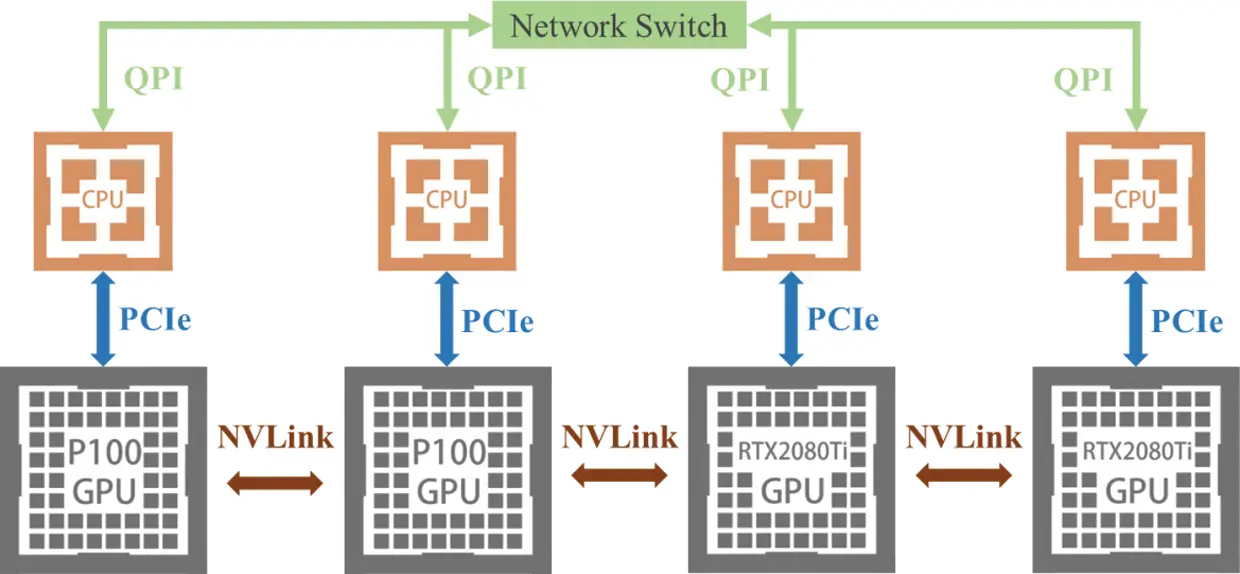Figure 9: GPU cluster workstation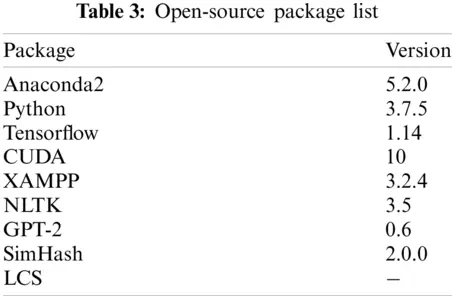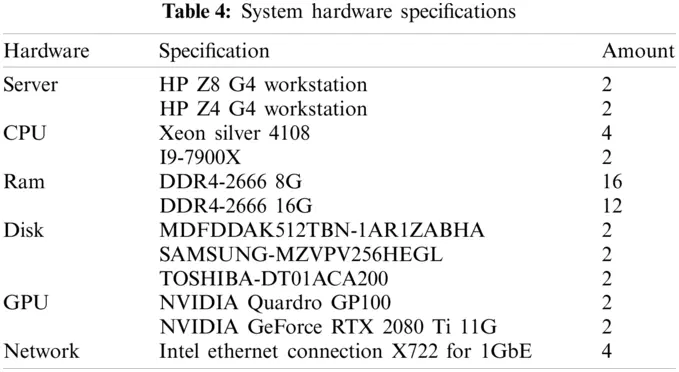4  Experimental Results and Discussion

4.1 Experimental Design

The experimental design is based on four example sentences in practice, and thus three experiments have carried out in this session. The experimental setting has proceeded word segmentation followed by keywords selection and sample program retrieval from semantic database. This study has acquired the sample programs from GitHub . The first experiment is to produce the newly generated GPT-2 based on every single sample program. The next is to check the code similarity and then verify the conformity of execution results between the generated program and the sample program. Finally, the last is to analyze the performance evaluation of the generated programs.

This study has built a semantic database for the experiments where keywords, sample program names, sample program paths, generated model paths, and other tables in the database created by XAMPP are shown in Fig. 10. Fig. 10 is a screenshot of the table of the four sample programs. In the following experiments, use these tables as training data sets. This session will also evaluate the improvement of the generated program's execution performance produced by code transform model GPT-2. In other words, this session would compare code lines and execution time between the generated program and the sample program based on two indicators (a) decreasing the percentage of code lines and (b) reduce the percentage of execution time.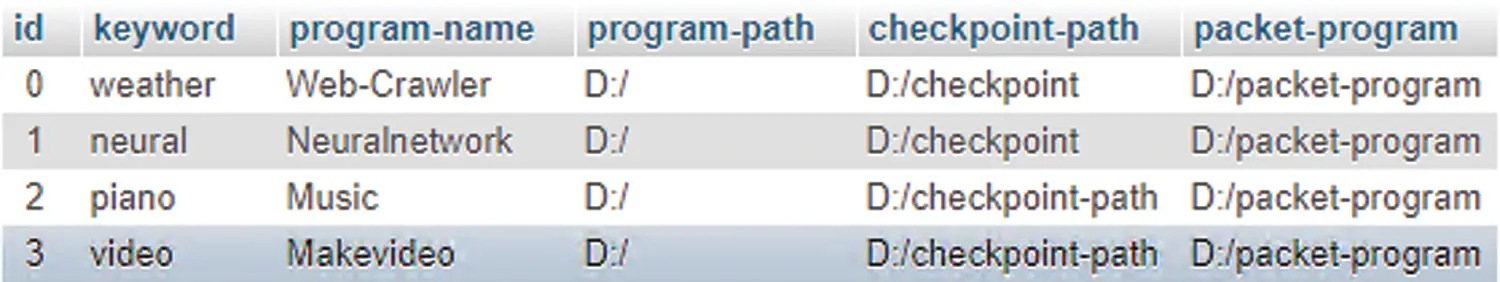Figure 10: Table of four sample programs

4.2 Experimental Settings

The experimental settings in this paragraph were divided into two parts. The first part was “selecting keywords for text segmentation and keyword search optimization.” In this research, four example sentences were used to optimize keyword retrieval by filtering redundancy and adding new keywords. Moreover, this research tested the accuracy difference between the original keyword search and the optimized keyword search. One example sentence corresponded to the keywords in different fields. The example sentences are shown in Tab. 5.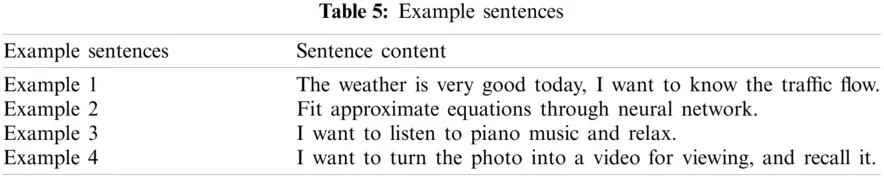The sample program of Example 1 is related to a web crawler , and the corresponding keywords were “weather, traffic”. The purpose of sample program 1 was to crawl the corresponding data on the Internet to get the weather forecast from Weather Center and automatically allocate the traffic congestion spots on Google Maps. Next, in the sample program of Example 2 the corresponding keywords are “equations, neural, network” related to the application of neural network . The main purpose of the sample program was to find approximate equations by training neural networks. Third, in the sample program of Example 3, the corresponding keyword is “piano, music,” and it is related to the program generating music . The objective of web camera programming was to generate a short piece of piano music automatically. Finally, the corresponding keywords of the sample program of Example 4 are “photo, video”. The program can turn photos into videos for users to watch .

The above four sentences were used as the word segmentation model NLTK to select the keywords. The results of keyword selection are shown in Tab. 6. The screenshot is shown in Fig. 11.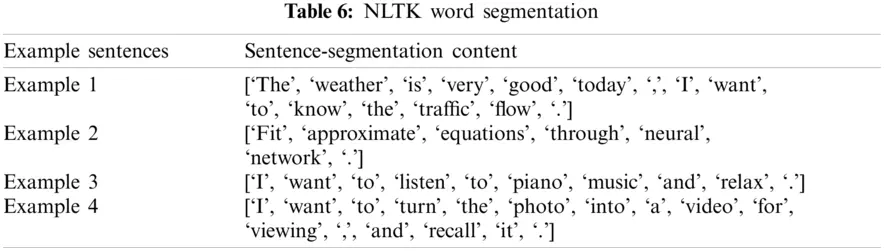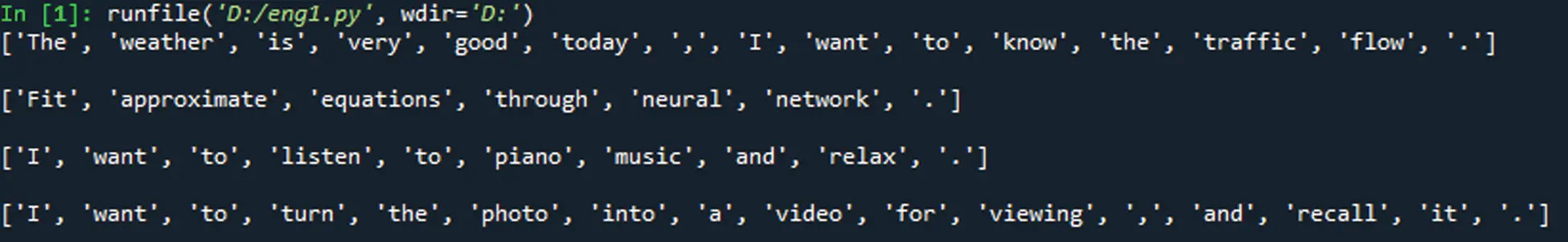Figure 11: Screenshot of NLTK word segmentation

In an unoptimized experiment, NLTK carried out word segmentation. Select all segmented words as keywords except punctuation. The chosen keywords are shown in Tab. 7, and the screenshot is shown in Fig. 12.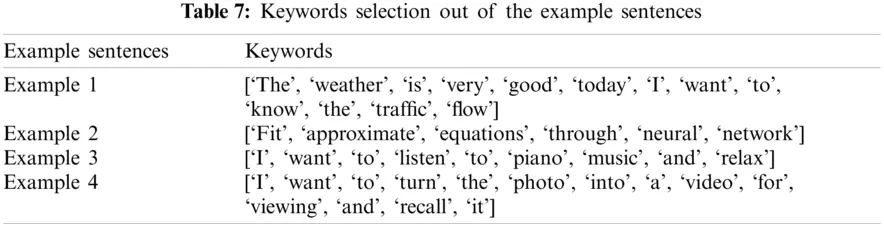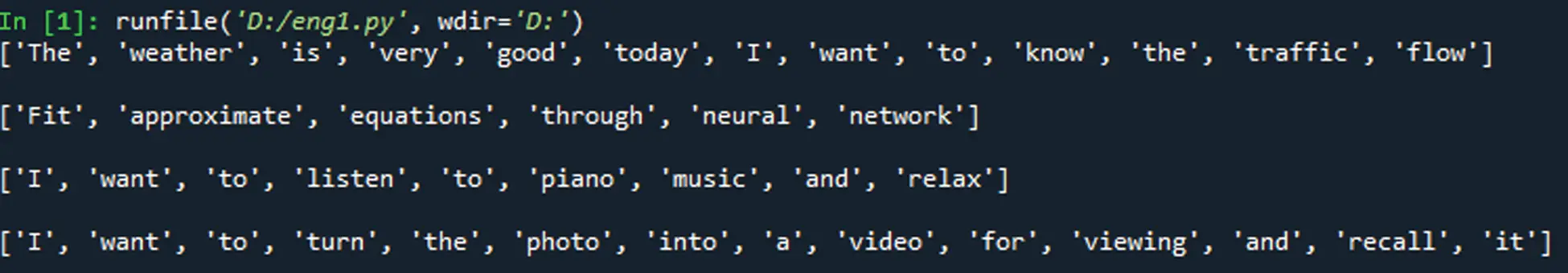Figure 12: Screenshot of selected keywords

The original keywords were consistent with the keywords in the semantic database, as shown in Tab. 8. Hit keywords were “weather, traffic” in Example 1, “equations, neural, network” in Example 2, “piano, music” in Example 3, and “photo, video” in Example 4.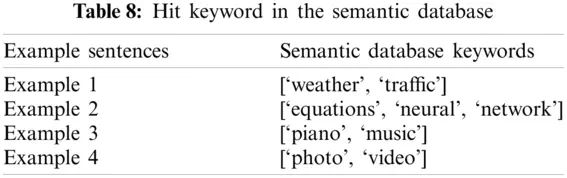4.3 Experimental Results

4.3.1 Experiment 1

The first experiment was based on four sample programs in GitHub. Corresponding to the keywords in the first experiment, extract the corresponding keywords from the sample program's natural language sentences. Besides, programs were generated in the system. The correspondence and purpose of the sample programs and keywords are described in Tab. 9 below.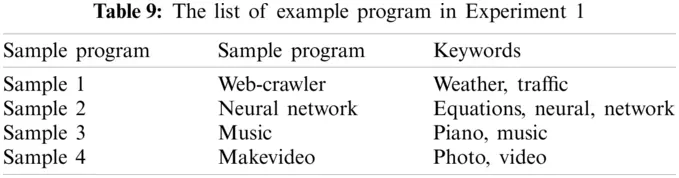To transform sample programs to the high-performance generated programs, a code transform model GPT-2 generated 100 preliminary programs, and its time consuming was also recorded at the same time. In this experiment, a total of five rounds and the estimated average time to generate a program in real-time was summary in Tab. 10.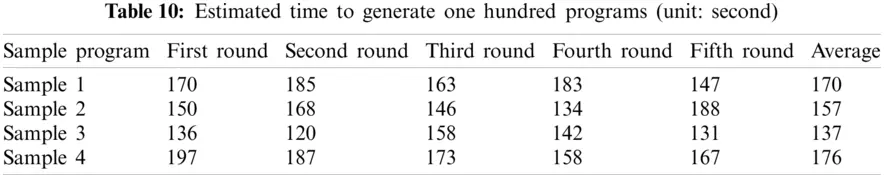4.3.2 Experiment 2

In experiment 2, the comparison of similarity between the above four sample programs and perform the program generated by GPT-2 on a cluster GPU workstation where samples of the generated programs are shown in Appendix. The purpose was to determine how many completed programs would have a similarity percentage greater than or equal to the users’ default passing ratio. In this experiment, the diagram of qualified ratio distribution set the X-axis as the similarity percentage, ranging from 0% to 100% with 20% as the separation interval. Set the Y-axis as the number of programs in the percentage ratio interval. The generated programs’ pass ratios are shown in Fig. 13. The pass ratio specified in this experiment was how many programs in 100 programs would have the similarity falling within the range of 80%∼100%. In other words, the passing ratios of the generated programs of Sample 1, 2, 3, and 4 were respectively 40%, 35%, 31%, and 32%.Figure 13: The pass ratio of the preliminary programs associated with each program in Experiment 2 (a) sample program 1 (b) sample program 2 (c) sample program 3 (d) sample program 4

4.3.3 Experiment 3

The fourth experiment first devotes to verify whether the generated program's execution result meets a certain proportion of conformity with the sample program. After Simhash similarity screening and generated programs compiled successfully, select the qualified programs. Then the qualified program with the highest pass ratio and the corresponding sample program are executed individually. Their execution results are shown in Figs. 1417. The program execution result is converted into ASCII code or binary code through LCS algorithm to compare it. The experimental result is listed as shown in Tab. 11.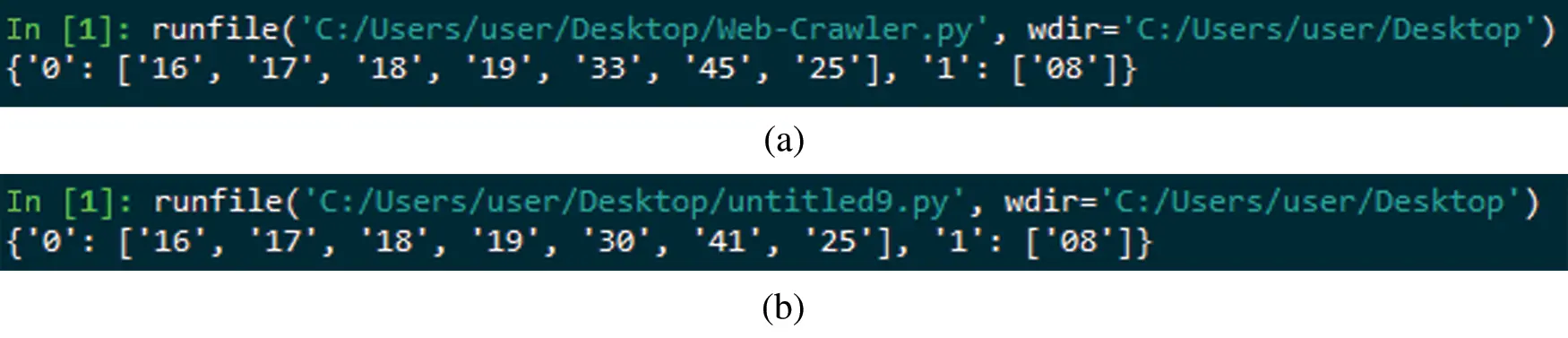Figure 14: Program execution result in Example 1 (a) Execution result of sample program 1 (b) Execution result of the best-qualified program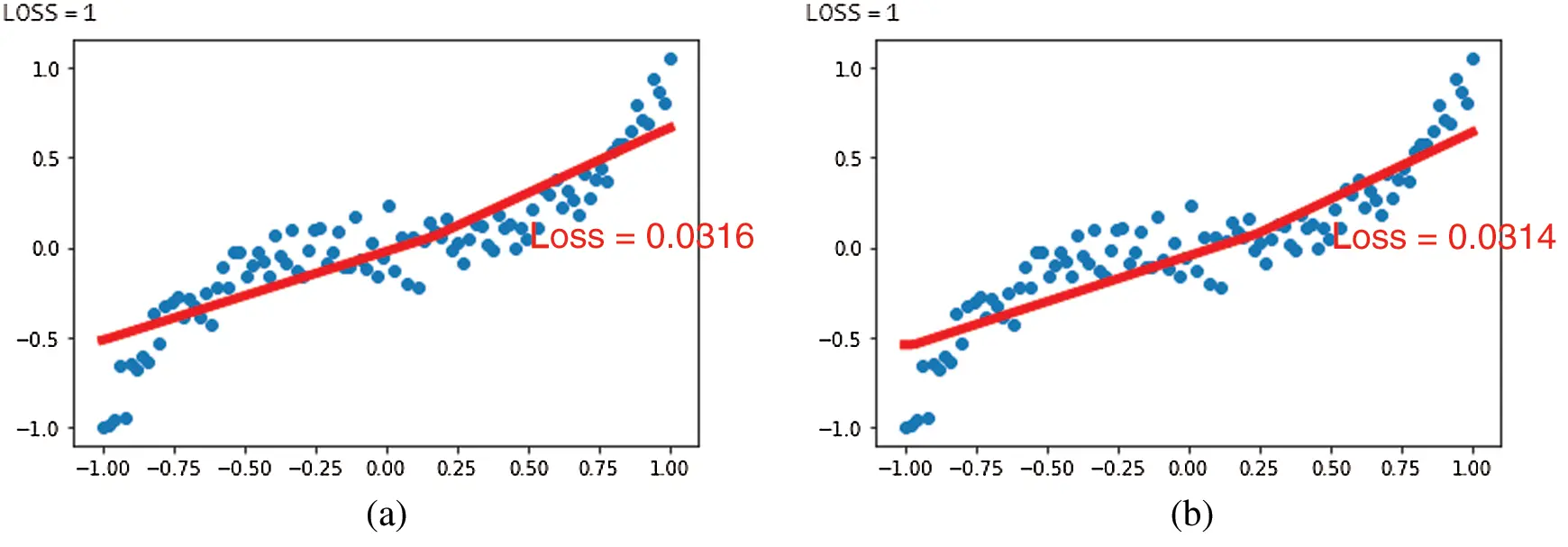Figure 15: Program execution result in Example 2 (a) Execution result of sample program 2 (b) Execution result of the best-qualified program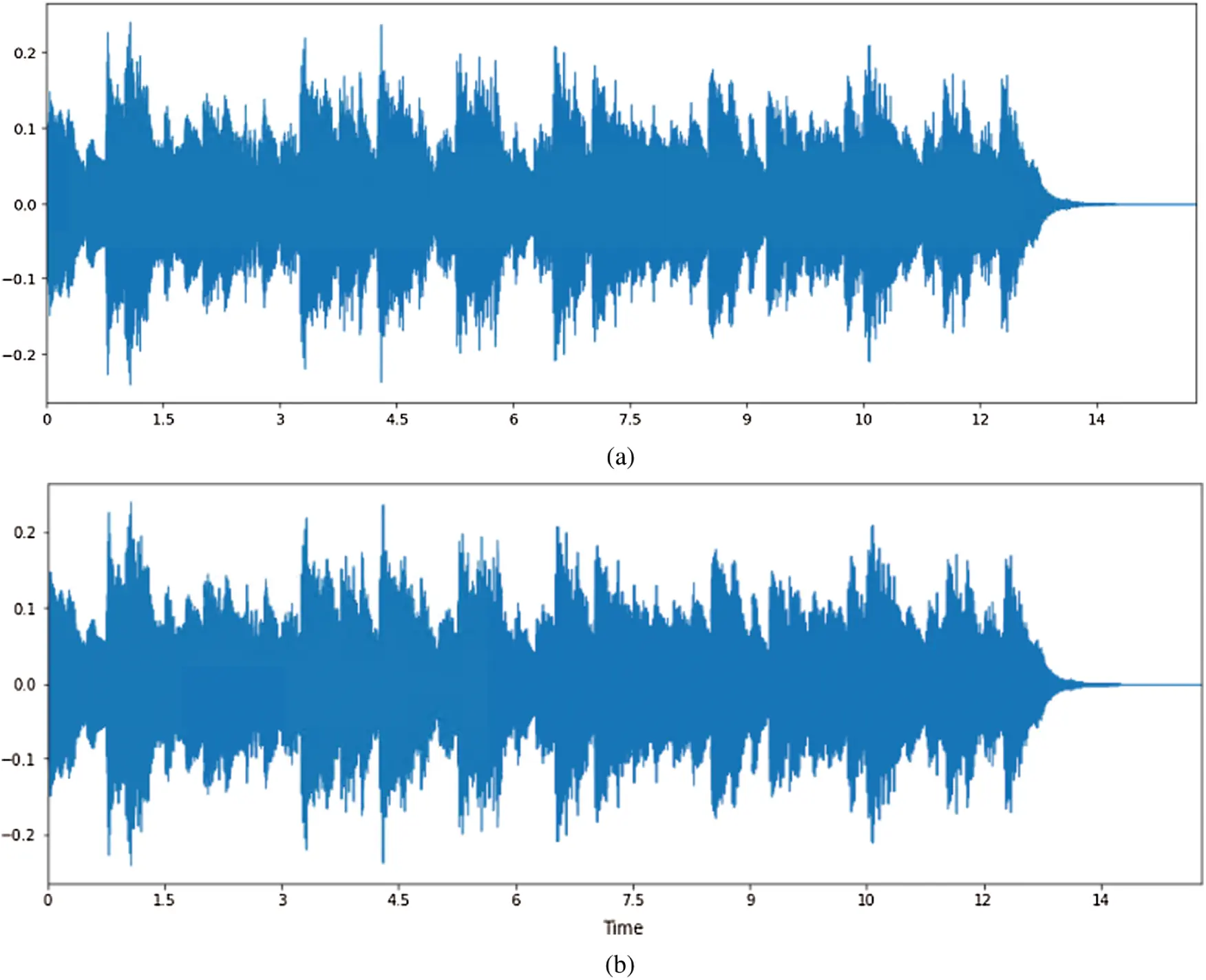Figure 16: Program execution results in Example 3 (a) Execution result of sample program 3 (b) Execution result of the best-qualified program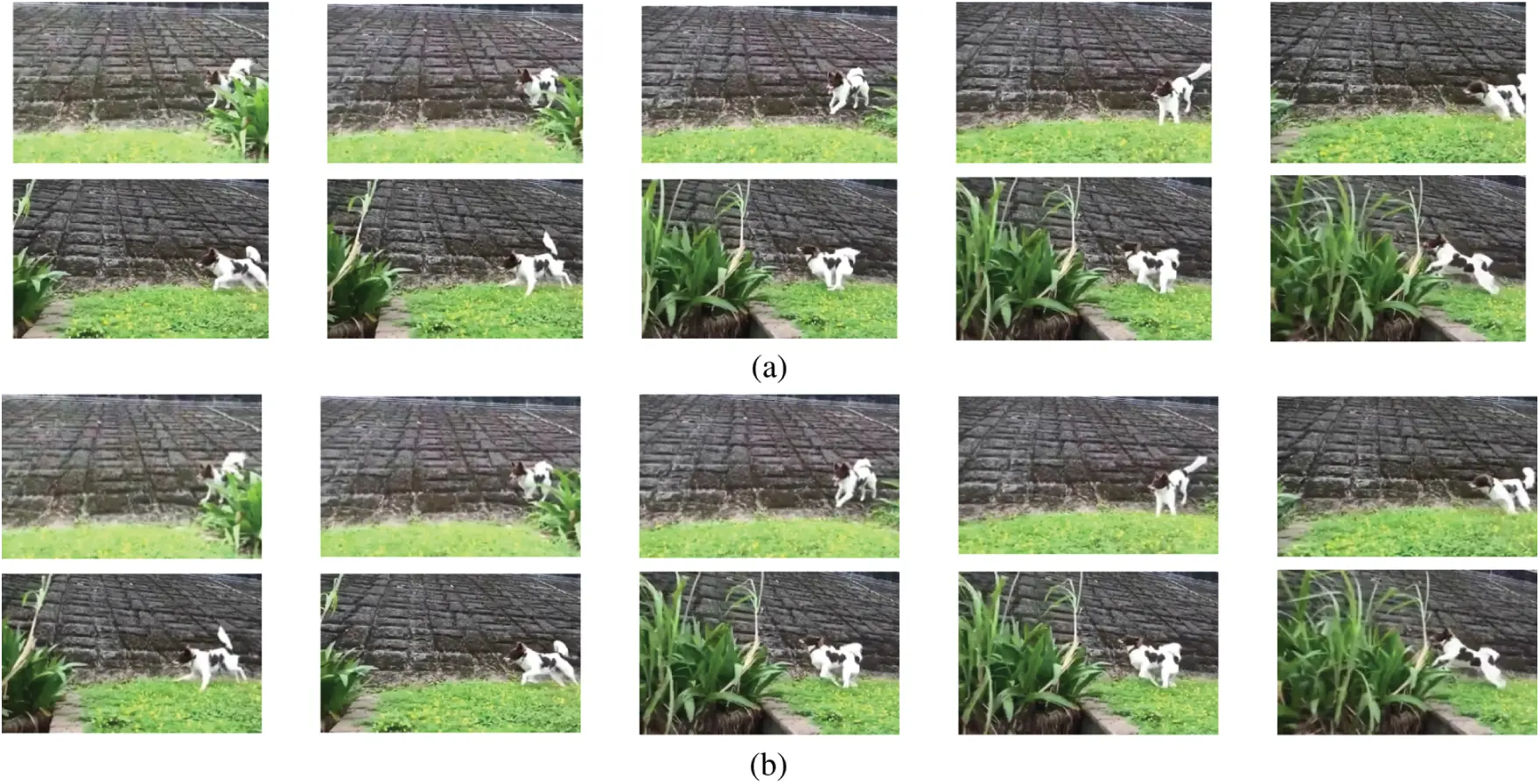Figure 17: Program execution results in Example 4 (a) Execution result of sample program 4 (capturing 10 consecutive frames) (b) Execution result of the best-qualified program (capturing ten consecutive frames)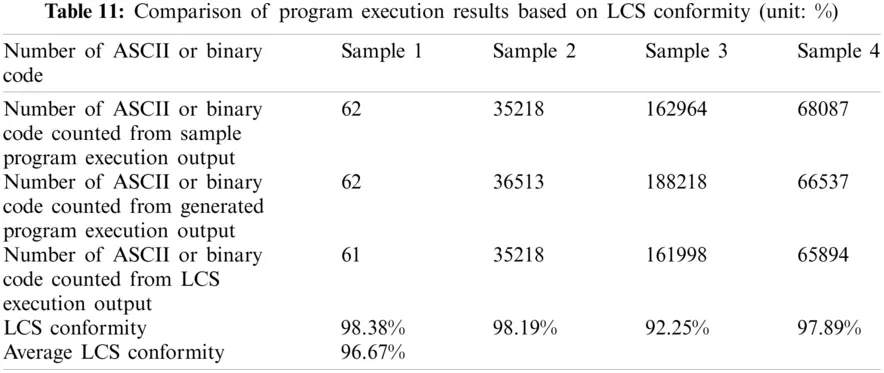Next, this experiment compared the performance of the above four generated programs produced by GPT-2 with the sample programs. The performance evaluation includes (a) comparing the number of code lines between the sample program and the generated program, and (b) the comparison comparing the execution time of the sample program and the generated program. To understand how much it speeds up program execution. The average number of code lines of 100 generated programs and the average execution time for those generated programs were carefully examined. The experimental results are listed in Tabs. 12 and 13, respectively.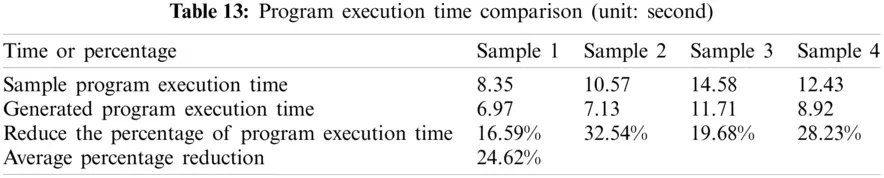4.4 Discussion

In the first experiment, based on the predetermined number of generated programs statistically, GPT-2 can produce 100 preliminary programs successfully for every corresponding sample program, that is, it takes approximately 1.6 s to generate a single prelimnary program. Furthermore, the newly generated program resulted in the code lines reduction where every generated preliminary program has roughly reduced 27.21% of code line in a single program. Therefore, the program execution time could be shortened possibly. After the code similarity checking between the preliminary program and sample program using variational Simhash algorithm, the second experiment selected a few preliminary programs (around 34.5%) with a higher pass ratio, thus called qualified programs. In other words, this screening process has filtered out 65 unqualified programs. Finally, once the qualified programs have been compiled successfully, the last experiment has proceeded with the execution result checking between qualified programs and sample program using Piecewise LCS algorithm. This verification has achieved the conformity 97.60% on average for four examples and we have selected the qualified program with the best comformity as a pocket program. Furthermore, the program execution time is reduced by 24.62% in the run time test, which implies that program execution performance has improved significantly. The experimental results shows that the proposed approach is with the creditability and validity.

5  Conclusion

This study has introduced an approach to effectively producing the newly generated programs using GPT-2. In addition, this study has proposed variational Simhash algorithm and piecewise longest common subsequence to verify the newly generated programs so as to achieve a single qualified higher efficiency computer program that can produce the correct execution result. According to the tests of four uses cases, the average number of newly generated code lines decreased by 27.21%. The average execution time of the program decreased by 24.62%. As a result, the proposed approach can quickly generate new programs that outperform the corresponding sample programs.

Data Availability: The Sample Program.zip data used to support this study's findings have been deposited in the https://drive.google.com/file/d/1wiG321g6p-Cq1J0Eca64IBNUHoTzjVGl/view?usp=sharing repository. The sample sentence data used to support the findings of this study are included within the article.

Author Contributions: B.R.C. and P.W.S. conceived and designed the experiments; H. F. T. collected the experimental dataset and proofread the paper; B. R.C. wrote the paper.

Funding Statement: This work is fully supported by the Ministry of Science and Technology, Taiwan, Republic of China, under Grant Nos. MOST 110-2622-E-390-001 and MOST 109-2622-E-390-002-CC3.

Conflicts of Interest: The authors declare that they have no conflicts of interest to report regarding the present study.

## References

1. Sampson, J. R. (2006). Artificial intelligence. SIAM Review, 18, 784-786. [Google Scholar] [CrossRef]
2. Yan, X., Mou, L., Li, G., Chen, Y., Peng, H. et al. (2015). Classifying relations via long short term memory networks along shortest dependency path. arXiv preprint arXiv: 1508.03720.
3. Zhao, J., Mathieu, M., LeCun, Y. (2016). Energy-based generative adversarial network. arXiv preprint arXiv: 1609.03126.
4. Shah, H., Warwick, K., Vallverdú, J., & Wu, D. (2016). Can machines talk? comparison of eliza with modern dialogue systems. Computers in Human Behavior, 58, 278-295. [Google Scholar] [CrossRef]
5. Arora, S., Athavale, V. A., Maggu, H., Agarwal, A. (2021). Artificial intelligence and virtual assistant—working model. Mobile Radio Communications and 5G Networks, pp. 163–171. Singapore: Springer.
6. Wagner, W., Bird, S., Klein, E., & Loper, E. (2010). Natural language processing with python, analyzing text with the natural language toolkit. Language Resources and Evaluation, 44(4), 421-424. [Google Scholar] [CrossRef]
7. Radford, A., Narasimhan, K., Salimans, T., Sutskever, I. (2019). Improving language understanding by generative pre-training. https://s3-us-west-2.amazonaws.com/openai-assets/research-covers/language-unsupervi-sed/language_understanding_paper.pdf.
8. Zamora, I., Lopez, N. G., Vilches, V. M., Cordero, A. H. (2016). Extending the openai gym for robotics: A toolkit for reinforcement learning using ros and gazebo. arXiv preprint arXiv: 1608.05742.
9. Lazer, D. M., Baum, M. A., Benkler, Y., Berinsky, A. J., & Greenhill, K. M. (2018). The science of fake news. Science, 359(6380), 1094-1096. [Google Scholar] [CrossRef]
10. Millman, K. J., & Aivazis, M. (2011). Python for scientists and engineers. Computing in Science & Engineering, 13, 9-12. [Google Scholar] [CrossRef]
11. Chang, B. R., Tsai, H. F., & Su, P. W. (2021). Applying code transform model to newly generated program for improving execution performance. Scientific Programming, 2021, 21. [Google Scholar] [CrossRef]
12. Cui, Y., Ahmad, S., & Hawkins, J. (2016). Continuous online sequence learning with an unsupervised neural network model. Neural Computation, 28, 2474-2504. [Google Scholar] [CrossRef]
13. Myagmar, B., Li, J., & Kimura, S. (2019). Cross-domain sentiment classification with bidirectional contextualized transformer language models. IEEE Access, 7, 163219-163230. [Google Scholar] [CrossRef]
14. Schoenmackers, S., Davis, J., Etzioni, O., Weld, D. (2010). Learning first-order horn clauses from web text. Proceedings of the 2010 Conference on Empirical Methods on Natural Language Processing, pp. 1088–1098. MIT Stata Center, Massachusetts, USA.
15. Gilbert, E. (2013). Widespread underprovision on reddit. Proceedings of the 2013 Conference on Computer Supported Cooperative Work, pp. 803–808. San Antonio, Texas, USA.
16. Alammar, J. (2018). The illustrated transformer. https://jalammar.github.io/illustrated-transformer/.
17. Over, D. E., Hadjichristidis, C., Evans, J. S. B., Handley, S. J., & Sloman, S. A. (2007). The probability of causal conditionals. Cognitive Psychology, 54(1), 62-97. [Google Scholar] [CrossRef]
18. Flaminio, T., Godo, L., & Hosni, H. (2020). Boolean algebras of conditionals. Artificial Intelligence, 286, 103347. [Google Scholar] [CrossRef]
19. Dvorski, D. D. (2007). Installing, configuring, and developing with XAMPP. Skills Canada.
20. Park, J. S., Chen, M. S., & Yu, P. S. (1997). Using a hash-based method with transaction trimming for mining association rules. IEEE Transactions on Knowledge and Data Engineering, 9(5), 813-825. [Google Scholar] [CrossRef]
22. Jain, A. K., & Feng, J. (2010). Latent fingerprint matching. IEEE Transactions on Pattern Analysis and Machine Intelligence, 33, 88-100. [Google Scholar] [CrossRef]
23. Zhang, L., Zhang, Y., Tang, J., Lu, K., Tian, Q. (2013). Binary code ranking with weighted hamming distance. Proceedings of the IEEE Conference on Computer Vision and Pattern Recognition, pp. 1586–1593. San Juan, PR, USA.
24. Schulz, J. (2008). Hamming distance. http://www.code10.info/index.php%3Foption%3Dcom_content%26view%3Darticle%26id%3D59:hamming-distance%26catid%3D38:cat_coding_algorithms_data-similarity%26Itemid%3D57.
25. Chou, H. L. (2021). Test1. https://github.com/m1085504/Data-exsaple/blob/main/test.
26. Chou, H. L. (2021). Test2. https://github.com/m1085504/Data-exsaple/blob/main/test1.
27. Burghardt, J. (2021). Longest common subsequence problem. https://en.wikipedia.org/wiki/Longest_common_subsequence_problem.
28. Tiedemann, J. (1999). Automatic construction of weighted string similarity measures. Proceedings of Joint SIGDAT Conference on Empirical Methods in Natural Language Processing and Very Large Corpora, pp. 213–219. College Park, MD, USA.
29. Chou, H. L. (2021). Exchange-rate. https://github.com/m1085504/Data-exsaple/blob/main/Exchange-Rate.
30. Chou, H. L. (2021). Picture. https://github.com/m1085504/Data-exsaple/blob/main/picture.
31. Chou, H. L. (2021). Voice. https://github.com/m1085504/Data-exsaple/blob/main/voice.
32. Chou, H. L. (2021). Video. https://github.com/m1085504/Data-exsaple/blob/main/video.
33. Cai, Y., Li, Y., Qiu, C., Ma, J., & Gao, X. (2019). Medical image retrieval based on convolutional neural network and supervised hashing. IEEE Access, 7, 51877-51885. [Google Scholar] [CrossRef]
34. Song, X., Tian, P., Yang, Y. (2012). Recognition of live performance sound and studio recording sound based on audio comparison. 2012 3rd IEEE International Conference on Network Infrastructure and Digital Content, pp. 21–23. Beijing, China.
35. Arndt, T., Chang, S. K. (1989). Image sequence compression by iconic indexing. 1989 IEEE Workshop on Visual Languages, pp. 177–182. The Institute of Electrical and Electronic Engineers, IEEE Computer Society, Silverspring, MD. DOI 10.1109/WVL.1989.77061. [CrossRef]
36. Redmon, J. (2019). YOLO: Real time object detection. https://github.com/pjreddie/darknet/wiki/YOLO:-Real-Time-Object-Detection.
37. Bouache, M., Glover, J. L., Boukhobza, J. (2016). Analysis of memory performance: mixed rank performance across microarchitectures. International Conference on High Performance Computing, pp. 579–590. Cham, Springer.
38. Foley, D., & Danskin, J. (2017). Ultra-performance pascal GPU and NVLink interconnect. IEEE Micro, 37(2), 7-17. [Google Scholar] [CrossRef]
39. Kalliamvakou, E., Gousios, G., Blincoe, K., Singer, L., German, D. M. et al. (2014). The promises and perils of mining github. Proceedings of the 11th Working Conference on Mining Software Repositories, pp. 92–101. Hyderabad, India.
40. Lin, J. W. (2020). Web-crawler. https://github.com/jwlin/web-crawler-tutorial.
41. Chou, H. L. (2021). Network. https://github.com/m1085504/Data-exsaple/blob/main/Network.
42. Chou, H. L. (2021). Music. https://github.com/m1085504/Data-exsaple/blob/main/Mucis.
43. Chou, H. L. (2021). Makevideo. https://github.com/m1085504/Data-exsaple/blob/main/Makevideo.

Appendix

In the Experiment 2, samples of the generated programs are shown in Figs. 1821.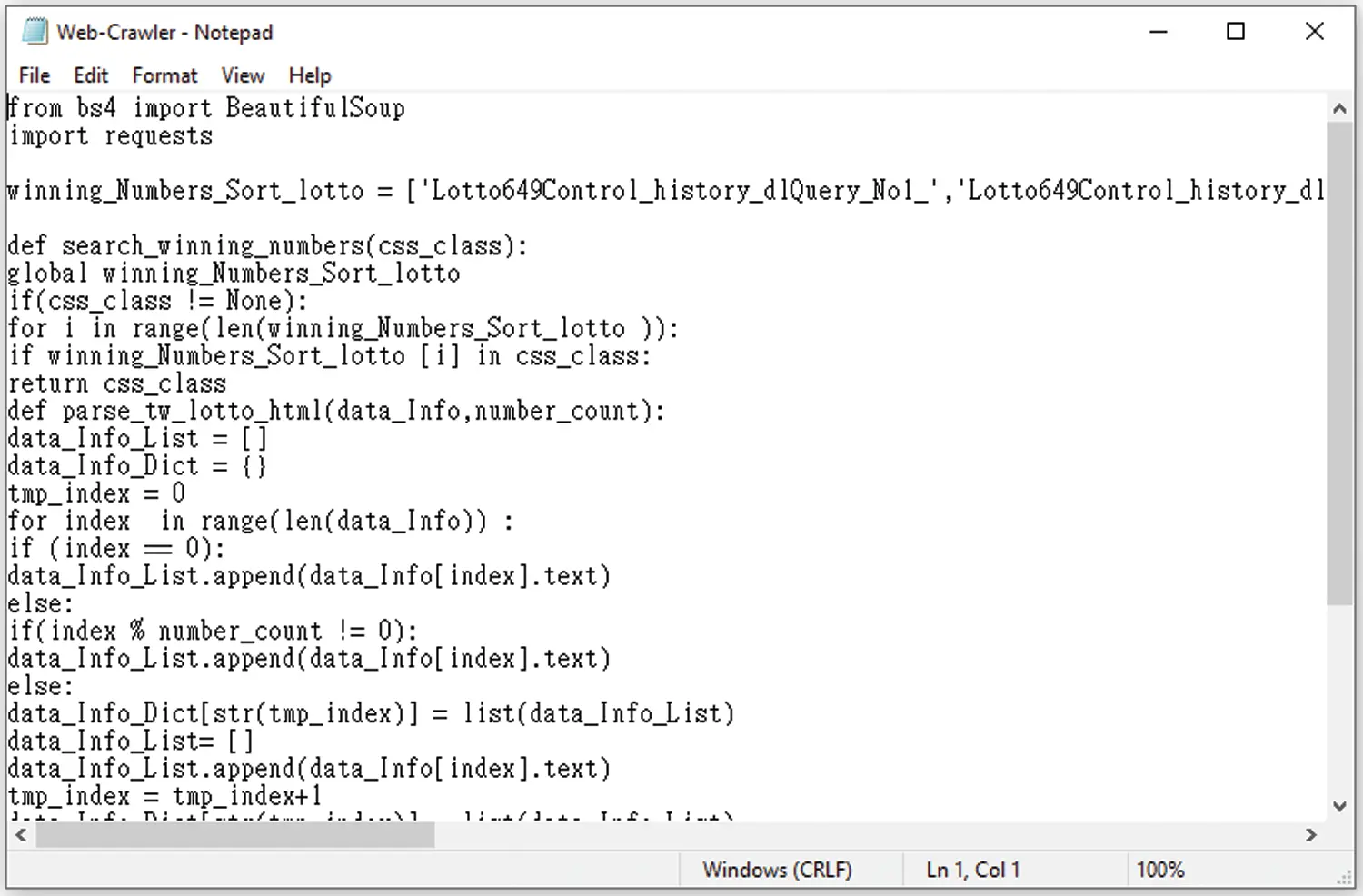Figure 18: Sampled preliminary program associated with sample program 1 in Experiment 2Figure 19: Sampled preliminary program associated with sample program 2 in Experiment 2Figure 20: Sampled preliminary program associated with sample program 3 in Experiment 2Figure 21: Sampled preliminary program associated with sample program 4 in Experiment 2This work is licensed under a Creative Commons Attribution 4.0 International License, which permits unrestricted use, distribution, and reproduction in any medium, provided the original work is properly cited.